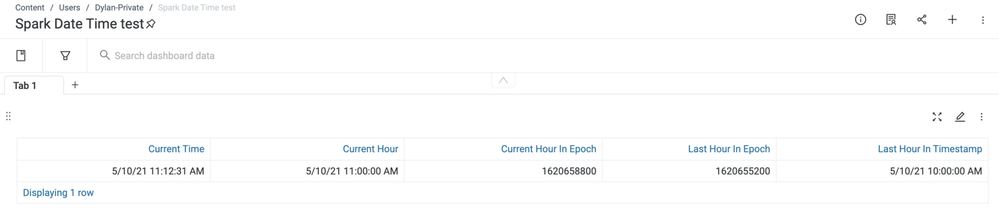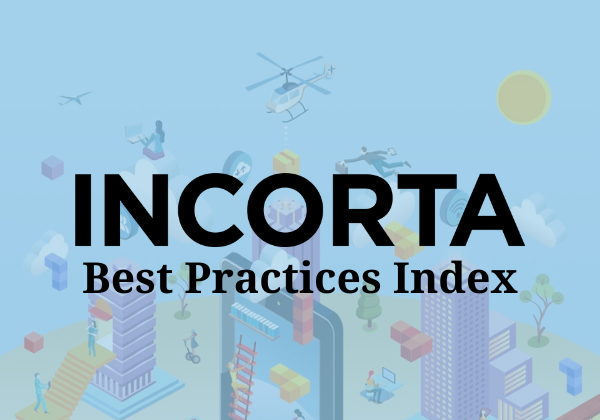cancel
Showing results for
Did you mean:

## How to handle Date Time in Spark MVEmployee

We would like to get the current hour, how can we get it in Spark SQL?   We also need the time as of one hour ago, how do we do that?

Here is a sample code that shows how we can produce it using Spark.  There is more than one way to do it.

We can use epoch time as the bridge for doing many calculations.

```df = spark.sql('''
SELECT  current_timestamp() CURRENT_TIME,
DATE_TRUNC('HOUR',current_timestamp()) CURRENT_HOUR,
CAST(DATE_TRUNC('HOUR',current_timestamp()) AS LONG) CURRENT_HOUR_IN_EPOCH,
CAST(DATE_TRUNC('HOUR',current_timestamp()) AS LONG) - 60*60 LAST_HOUR_IN_EPOCH,
timestamp(CAST(DATE_TRUNC('HOUR',current_timestamp()) AS LONG) - 60*60 ) LAST_HOUR_IN_TIMESTAMP
''')

save(df)```

It shows results:Best Practices IndexJust here to browse knowledge? This might help!

Contributors
Version history
Last update:
‎04-07-2022 12:23 PM
Updated by: